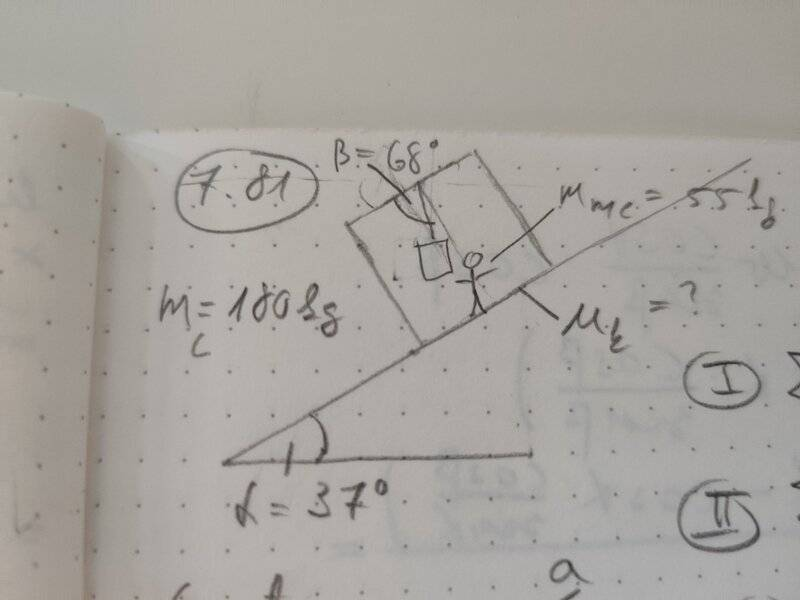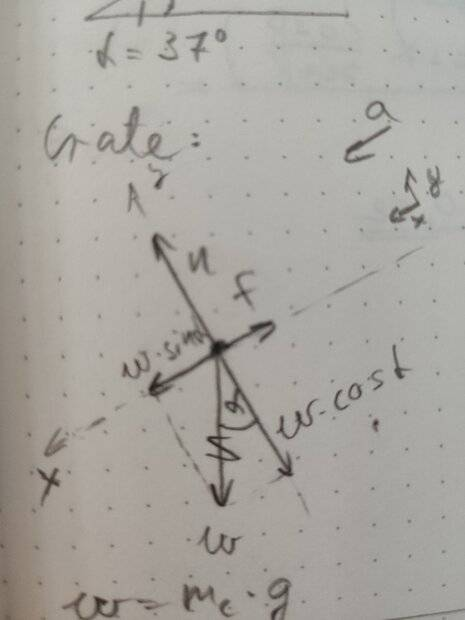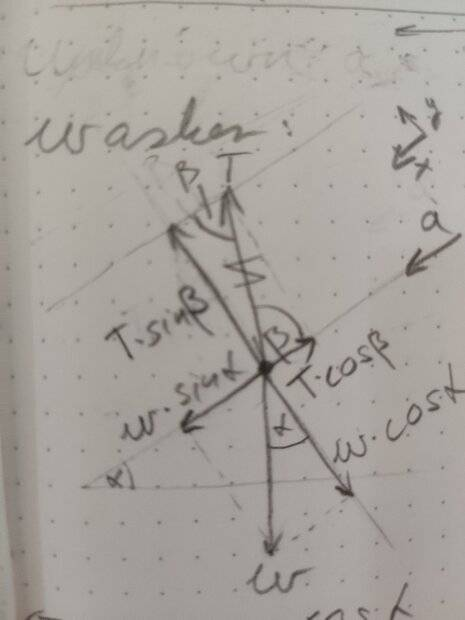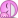# Find the Coefficient of Kinetic Friction

Argonaut
Homework Statement:
A steel washer is suspended inside an empty shipping crate from a light string attached to the top of the crate. The crate slides down a long ramp that is inclined at an angle of 37° above the horizontal. The crate has mass 180 kg. You are sitting inside the crate(with a flashlight); your mass is 55 kg. As the crate is sliding down the ramp, you find the washer is at rest with respect to the crate when the string makes an angle of 68° with the top of the crate. What is the coefficient of kinetic friction between the ramp and the crate?
Relevant Equations:
Newton's First and Second Laws
Young & Freedman 13th ed, Exercise 7.81Starting with the crate, here is its free-body diagram:In accordance with Newton's First Law:

$$\Sigma F_y = 0 = n+(-w_c \cos{\alpha})$$

Thus ## n = w_c \cos{\alpha} ##.

And according to Newton's Second Law:

$$\Sigma F_x = m_c a_x = w_c \sin{\alpha} + (-f)$$

Utilising the fact that ## f = \mu_k n ## and substituting n with the expression above, we get:

\begin{align*} m_c a_x &= w_c \sin{\alpha} - \mu_k n \\ &= w_c \sin{\alpha} - \mu_k w_c \cos{\alpha} \\ &= m_c g (\sin{\alpha} - \mu_k \cos{\alpha}) \end{align*}

Thus ## \mu_k = \frac{\sin{\alpha} - a_x/g}{\cos{\alpha}} ##.

##a_x## is unknown. Switching to the washer, it's free-body diagram:According to Newton's First Law:

$$\Sigma F_y = 0 = T \sin{\beta} - w_w \cos{\alpha}$$

Thus we have ## T = w_w \frac{\cos{\alpha}}{\sin{\beta}} ##.

According to Newton's Second Law:

$$\Sigma F_x = m_w a_x = w_w \sin{\alpha} -T \cos{\beta}$$

Substituting T with the expression above and rearranging to express ## a_x ##, we get:

\begin{align*} m_w a_x &= w_w \sin{\alpha} -T \cos{\beta} \\ &= w_w \sin{\alpha} - w_w \frac{\cos{\alpha}}{\sin{\beta}} \cos{\beta} \end{align*}

Thus ## a_x = g( \sin{\alpha} - \frac{\cos{\alpha}}{\sin{\beta}} \cos{\beta}) ##

Plugging in ##a_x##to the expression for ## \mu_k##, we get:

\begin{align*} \mu_k &= \sin{\alpha}( \sin{\alpha} - \frac{\cos{\alpha}}{\sin{\beta}} \cos{\beta})/\cos{\alpha} \\ &= \frac{\cos{\beta}}{\sin{\alpha}} \\ &= \frac{\cos{68°}}{\sin{37°}} \\ &\approx 0.62 \end{align*}

The book solution says it's 0.40. Where did I make a mistake?

Homework Helper
Gold Member
2022 Award
Plugging in ##a_x##to the expression for ## \mu_k##, we get:
Check your algebra for 'plugging in' and what follows.

Also, you have used the mass and weight of the crate alone in various equations – but you should have used totals (crate + person). However, since ##m## ans ##w## cancel-out in the various equations, it shouldn’t affect the answer.

••PeroK and Argonaut
Argonaut
\begin{align*} \mu_k &= \sin{\alpha}-( \sin{\alpha} - \frac{\cos{\alpha}}{\sin{\beta}} \cos{\beta})/\cos{\alpha} \\ &= \frac{\cos{\beta}}{\sin{\alpha}} \\ &= \frac{\cos{68°}}{\sin{37°}} \\ &\approx 0.62 \end{align*}

The book solution says it's 0.40. Where did I make a mistake?

Don't mind me - I've found it. I made a mistake while doing the algebraic manipulation at the end. Very silly. (And there was a mistake in my LaTeX too).
The correct seqence:

\begin{align*} \mu_k &= (\sin{\alpha}-( \sin{\alpha} - \frac{\cos{\alpha}}{\sin{\beta}} \cos{\beta}))/\cos{\alpha} \\ &= \frac{\cos{\beta}}{\sin{\beta}} \\ &= \frac{\cos{68°}}{\sin{68°}} \\ &\approx 0.40 \end{align*}

Lesson: Don't skip steps - better to write everything out if you are bad at mental arithmetic.

•Steve4Physics
Argonaut
Check your algebra for 'plugging in' and what follows.

Also, you have used the mass and weight of the crate alone in various equations – but you should have used totals (crate + person). However, since ##m## ans ##w## cancel-out in the various equations, it shouldn’t affect the answer.
Thanks!

About the mass of the crate, the exercise says "The crate has mass 180 kg". I assumed it meant the crate including its content, so the person and the washing machine. Do you think they meant the empty crate? Even if as you say, it's moot as the mass cancels out.

Homework Helper
Gold Member
2022 Award
Thanks!

About the mass of the crate, the exercise says "The crate has mass 180 kg". I assumed it meant the crate including its content, so the person and the washing machine. Do you think they meant the empty crate? Even if as you say, it's moot as the mass cancels out.
The question gives separate values of mass for the crate and the person (180kg and 55kg). I interpret that as a total mass of 235kg. For example, if asked to calculate the frictional force, I would use 235kg in the calculation.

Note: A ‘washer’ in the context of this question is a small metal disc with a hole in the middle. E.g. see https://www.ssflatwashers.com/china...e_diameter_washer_clean_surface-11725011.html

You would not want to be trapped inside a crate, sliding down a hill, with a washing machine suspended by a 'light string' hanging over you! (Now I understand your Post #1 diagram!)

•SammyS and Argonaut
Argonaut
The question gives separate values of mass for the crate and the person (180kg and 55kg). I interpret that as a total mass of 235kg. For example, if asked to calculate the frictional force, I would use 235kg in the calculation.

Note: A ‘washer’ in the context of this question is a small metal disc with a hole in the middle. E.g. see https://www.ssflatwashers.com/china...e_diameter_washer_clean_surface-11725011.html

You would not want to be trapped inside a crate, sliding down a hill, with a washing machine suspended by a 'light string' hanging over you! (Now I understand your Post #1 diagram!)
Ah hahaha, I love it! It did seem like a quirky setupThanks for the feedback again.

•SammyS